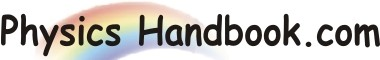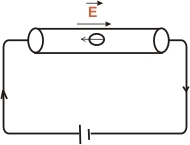HOME TOPICS DEFINITIONS TABLES LAWS INVENTIONS EXPERIMENTS QUIZ VIDEOS
 A B C D E F G H I J K L M N O P Q R S T U V W X Y Z
Electric Current

The flow of charge in define direction is called electric current. It is measured by the time rate of flow of charge through a conductor (or material). In the words, electric current is the flow of charge through any cross-section of the conductor per second. If q is the charge flowing through any cross-section of the conductor in time t, then,

Electric current, I = q / t

When a cell is connected across the ends of conductor (see the fig), electric field is set up in the conductor. The electric field exerts force on the free electrons, causing the free
Electrons to drift towards the positive terminal of the cell. This Constitutes electric current in the conductor. As convention the direction of flow of positive charge is taken as the direction of current flow. If moving charge are negative ,as with free flow of negative , as with free electrons in metal, then direction of current is opposite to the flow of negative charge. This convention does not make any difference in the actual operation of the electrical appliances.Note:
If the rate of flow of charge varies with time, then current at any time(i.e. instantaneous current) is given by;

i = dq / dt
where dq is a small charge passing through any cross-section of the conductor is small dt.

Unit of electric current:
The SI unit of charge is one coulomb and that of time is one second . Therefore, SI unit of current is 1 coulomb/sec which has been given a special name of ampere. If q = IC and t = 1s, then
I =1/1 = 1 ampere.

One ampere of current is said to flow through a wire if at any cross-section, one coulomb of charge flow in one second .
Thus, if 5 ampere current is flowing through a wire , it means that 5 C per second flows past any cross-section of the wire.

Note:
C = charge on 6.25×1018 electrons. Thus when we say that current through a wire is 1A, it means that 6.25×1018electrons per second flow past any cross-section of wire.
Therefore:

I = q / t = ne / t
where e = -1.6×10-19C